May 12, 2021, 02:45:21 PM

## News:

IonicWind Snippit Manager 2.xx Released!  Install it on a memory stick and take it with you!  With or without IWBasic!

## RF Current density on metal surface?

Started by aurelCB, November 16, 2017, 11:28:05 AM

0 Members and 1 Guest are viewing this topic.

#### aurelCB

#####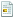November 16, 2017, 11:28:05 AM
Hi to all
my question may look confusing but in fact is simple.
I was looking all over internet for code ( in C,Basic etc) to how plot
electric current density on metal surface when current is RF ,
i found only some Matlab code but i dont understan how to translate such a code
to BASIC in this case CreativeBasic( still one of my favorite Basic dialects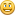)
So anyone GWS,Egil or someone else have idea about how to do that ?
Aurel
forum for hobby programming:<br />https://aurelsoft.ucoz.com

#### LarryMc

#####November 16, 2017, 01:03:29 PM #1
So what code did you find on Skin Depth theory on MatLab?  Or did you just find the equations without all the variables defined?
LarryMc
Larry McCaughn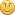Author of IWB+, Custom Button Designer library, Custom Chart Designer library, Snippet Manager, IWGrid control library, LM_Image control library

#### Egil

#####November 16, 2017, 01:56:22 PM #2
Interesting problem you present. I have never done such things myself, but have seen others doing it using MatLab. And instead of just simulating antenna theory I have found it more satifying to experiment with the real thing.

Maybe this is the MatLab example you have found:   https://se.mathworks.com/help/antenna/examples/current-visualization-on-the-antenna-surface.html?s_tid=gn_loc_drop

The formulas found there should be possible to translate to any other programming language, including CB and IWB. But since I am not familiar with MatLab, and do not know the built-in commands, it is difficult to see  what more information you'll need to code in CB.
I know that one of the books referred to ( C. A. Balanis, 'Antenna Theory. Analysis and Design,' Wiley, New York, 3rd Edition, 2005. ) is regarded as kind of a "bible" for UHF/EHF antenna work. Guess the price will be high, but maybe it is available at a technical library in your country? I think all info you need to code your own program can be found in there.

Good Luck!
Egil

Support Amateur Radio  -  Have a ham  for dinner!

#### aurelCB

#####November 16, 2017, 02:22:02 PM #3
Hi Larry hi Egil
hmm i found something written in Python look:
i hope that can be translated to BASIC.

`import mathfrom math import cos, sin, sqrt, atan2, acosdef PatchFunction(thetaInDeg, phiInDeg, Freq, W, L, h, Er):    """    Taken from Design_patchr    Calculates total E-field pattern for patch as a function of theta and phi    Patch is assumed to be resonating in the (TMx 010) mode.    E-field is parallel to x-axis    W......Width of patch (m)    L......Length of patch (m)    h......Substrate thickness (m)    Er.....Dielectric constant of substrate    Refrence C.A. Balanis 2nd Edition Page 745    """    lamba = 3e8 / Freq    theta_in = math.radians(thetaInDeg)    phi_in = math.radians(phiInDeg)    ko = 2 * math.pi / lamba    xff, yff, zff = sph2cart1(999, theta_in, phi_in)                            # Rotate coords 90 deg about x-axis to match array_utils coord system with coord system used in the model.    xffd = zff    yffd = xff    zffd = yff    r, thp, php = cart2sph1(xffd, yffd, zffd)    phi = php    theta = thp    if theta == 0:        theta = 1e-9                                                              # Trap potential division by zero warning    if phi == 0:        phi = 1e-9    Ereff = ((Er + 1) / 2) + ((Er - 1) / 2) * (1 + 12 * (h / W)) ** -0.5        # Calculate effictive dielectric constant for microstrip line of width W on dielectric material of constant Er    F1 = (Ereff + 0.3) * (W / h + 0.264)                                        # Calculate increase length dL of patch length L due to fringing fields at each end, giving total effective length Leff = L + 2*dL    F2 = (Ereff - 0.258) * (W / h + 0.8)    dL = h * 0.412 * (F1 / F2)    Leff = L + 2 * dL    Weff = W                                                                    # Calculate effective width Weff for patch, uses standard Er value.    heff = h * sqrt(Er)    # Patch pattern function of theta and phi, note the theta and phi for the function are defined differently to theta_in and phi_in    Numtr2 = sin(ko * heff * cos(phi) / 2)    Demtr2 = (ko * heff * cos(phi)) / 2    Fphi = (Numtr2 / Demtr2) * cos((ko * Leff / 2) * sin(phi))    Numtr1 = sin((ko * heff / 2) * sin(theta))    Demtr1 = ((ko * heff / 2) * sin(theta))    Numtr1a = sin((ko * Weff / 2) * cos(theta))    Demtr1a = ((ko * Weff / 2) * cos(theta))    Ftheta = ((Numtr1 * Numtr1a) / (Demtr1 * Demtr1a)) * sin(theta)    # Due to groundplane, function is only valid for theta values :   0 < theta < 90   for all phi    # Modify pattern for theta values close to 90 to give smooth roll-off, standard model truncates H-plane at theta=90.    # PatEdgeSF has value=1 except at theta close to 90 where it drops (proportional to 1/x^2) to 0    rolloff_factor = 0.5                                                       # 1=sharp, 0=softer    theta_in_deg = theta_in * 180 / math.pi                                          # theta_in in Deg    F1 = 1 / (((rolloff_factor * (abs(theta_in_deg) - 90)) ** 2) + 0.001)       # intermediate calc    PatEdgeSF = 1 / (F1 + 1)                                                    # Pattern scaling factor    UNF = 1.0006                                                                # Unity normalisation factor for element pattern    if theta_in <= math.pi / 2:        Etot = Ftheta * Fphi * PatEdgeSF * UNF                                   # Total pattern by pattern multiplication    else:        Etot = 0    return Etot  def sph2cart1(r, th, phi):  x = r * cos(phi) * sin(th)  y = r * sin(phi) * sin(th)  z = r * cos(th)  return x, y, z  def cart2sph1(x, y, z):  r = sqrt(x**2 + y**2 + z**2) + 1e-15  th = acos(z / r)  phi = atan2(y, x)  return r, th, phi`
forum for hobby programming:<br />https://aurelsoft.ucoz.com

#### aurelCB

#####November 17, 2017, 11:57:08 AM #4
Hi Egil
Yes given link is fine but is not exactly what I (we) need.
There is presented ordinary dipole antenna with reflector but i need pattern
calculation for patch which is as you probably know rectangular piece of thin metal
copper or aluminum or brass.
what this pattern plot present is point where I must connect feed line or
coaxial cable ,in another words where (position) is the dark-red area is the best place to
create conntact, i hope that i dont bother you with all this stuff?
Patch or panel antenna as is dont have any great gain BUT
array of patches have very good gain up to 30dB
forum for hobby programming:<br />https://aurelsoft.ucoz.com

#### Egil

#####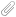November 18, 2017, 11:14:43 AM #5
Quotei hope that i dont bother you with all this stuff?

Not at all. But as I told you before, I have no experience with UHF/EHF antennas. And Panel antennas are mainly used for that frequencies.

QuoteYes given link is fine but is not exactly what I (we) need.
There is presented ordinary dipole antenna with reflector but i need pattern
calculation for patch which is as you probably know rectangular piece of thin metal
copper or aluminum or brass.

I think I misunderstood you at first, since you asked for RF current density along a  metal surface.
But whether an antenna is a dipole or any other type does not matter. The properties are always the same with a given material.
The impedance along the surface is a function of length in wavelengths from the point defined as ground. With an antenna made as a printed circuit board (such as Patch Antennas), the board material's electrical properties becomes a large part of these calculations.

I have no knowledge of the formulas needed for calculating the feedpoint of these antenna types, but remember that the german radio magazine "UKW Berichte" had a series of articles on the theory, and how to construct, panel antennas early in the 1970s. I beleive that magazine was also published in an english version, under the name of "VHF Communication".

I attach an article on Patch Antennas I found on one of my hardisks. I do not remember how it came in my posession, but hope the information can help you a little further with your work.

Egil

Support Amateur Radio  -  Have a ham  for dinner!

#### Egil

#####November 18, 2017, 12:47:20 PM #6
Zlatko,

I forgot to mention one of the best antenna web sites I've ever seen: http://www.antenna-theory.com.
The site has a very skillfull crowd in the user forum. So that is probably the best place to find the answer to your questions. And as soon as you have found the proper formulas, is should be easy converting  them to CB (or IWB) code.

I also found an article with a very good theoretical description of patch antennas on the site: http://www.antenna-theory.com/antennas/patches/antenna.php.

Good luck!
Egil.

PS: A quick Google search on "Patch Antenna Theory" gave more than 400 000 hits!

Support Amateur Radio  -  Have a ham  for dinner!

#### aurelCB

#####November 18, 2017, 01:36:36 PM #7
Hi Egil
and many thanks for informations
i will try first to convert this Python Code to BASIC to see is there any chance to work.
Why simply because code looking to me very clear.
of course i will visit sites you tell me
thanks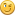forum for hobby programming:<br />https://aurelsoft.ucoz.com

#### aurelCB

#####April 23, 2019, 11:41:10 AM #8
Ok guys
after almost two years....time just flying ..
i tried to translate this but it looks that is too big problem for me ...
here is what i get but program simply crush...........

forum for hobby programming:<br />https://aurelsoft.ucoz.com

#### aurelCB

#####April 24, 2019, 12:05:35 AM #9
sorry guys because of stupid browser problems i don't post code
well i must say that i almost forget how to use Creative basic
probably because i use other tools(PL)  for programming...
i don't use python/matlab just found this one...

`'AutoDefine "Off"``def win:window``def wstyle:int``def cleft,ctop,cwidth,cheight:int :'Clientarea``def run:int``def edit1:int ``wstyle = @SIZE|@MINBOX|@MAXBOX``WINDOW win, 0,0,640,500,wstyle,0,"test", <MainLoop>``GETCLIENTSIZE win,cleft,ctop,cwidth,cheight``SETWINDOWCOLOR win,RGB(240,202,86)``CONTROL win,"E,"",10,10,100,20,0x50800000,10" :' Edit Control``'--------------------------------------------------------------------------------``'DIALOG d1,0,0,295,199,0x80C80080,0,"Caption",Handler``'CONTROL d1,"E,Edit1,31,44,70,20,0x50800000,1"``'PatchFunction(thetaInDeg:INT, phiInDeg:INT, Freq:INT, W:INT, L:INT, h:INT, Er:FLOAT``PatchFunction( 90, 120, 2400, 100, 40, 5, 0.7)``'-------------------------------------------------------------------------------``run=1``WAITUNTIL run = 0 ``CLOSEWINDOW win ``END ``'``SUB <MainLoop>``'-------------------------------------------------------------------------------------``SELECT @class`` CASE @idcreate `` centerwindow win `` CASE @idclosewindow `` run = 0 `` CASE @idsize`` GETCLIENTSIZE win,cleft,ctop,cwidth,cheight``    if CONTROLEXISTS(win,10)`` SETSIZE win,10,40,180,30,10``    endif ``ENDSELECT ``RETURN``'import math``'from math import cos, sin, sqrt, atan2, acos``SUB PatchFunction(thetaInDeg:INT, phiInDeg:INT, Freq:INT, W:INT, L:INT, h:INT, Er:FLOAT),INT``    ``    'Taken from Design_patchr``    'Calculates total E-field pattern for patch as a function of theta and phi``    'Patch is assumed to be resonating in the (TMx 010) mode.``    'E-field is parallel to x-axis``'    W......Width of patch (m)`` '   L......Length of patch (m)``  '  h......Substrate thickness (m)``  '  Er.....Dielectric constant of substrate``'    Refrence C.A. Balanis 2nd Edition Page 745`` '   """``  lamba = 3e8 / Freq``    theta_in = math.radians(thetaInDeg)``   phi_in = math.radians(phiInDeg)``    ko = 2 * math.pi / lamba``    xff, yff, zff = sph2cart1(999, theta_in, phi_in)            :' # Rotate coords 90 deg about x-axis to match array_utils coord system with coord system used in the model.``    xffd = zff``    yffd = xff``    zffd = yff``    r, thp, php = cart2sph1(xffd, yffd, zffd)``    phi = php``    theta = thp``    if theta = 0``        theta = 1e-9                                             :'# Trap potential division by zero warning``    if phi == 0:``        phi = 1e-9``    Ereff = ((Er + 1) / 2) + ((Er - 1) / 2) * (1 + 12 * (h / W)) ** -0.5  :'# Calculate effictive dielectric constant for microstrip line of width W on dielectric material of constant Er``    F1 = (Ereff + 0.3) * (W / h + 0.264)                                  :'# Calculate increase length dL of patch length L due to fringing fields at each end, giving total effective length Leff = L + 2*dL``    F2 = (Ereff - 0.258) * (W / h + 0.8)``    dL = h * 0.412 * (F1 / F2)``    Leff = L + 2 * dL``    Weff = W                                                              :'# Calculate effective width Weff for patch, uses standard Er value.``    heff = h * sqrt(Er)``   ' # Patch pattern function of theta and phi, note the theta and phi for the function are defined differently to theta_in and phi_in``    Numtr2 = sin(ko * heff * cos(phi) / 2)``    Demtr2 = (ko * heff * cos(phi)) / 2``    Fphi = (Numtr2 / Demtr2) * cos((ko * Leff / 2) * sin(phi))``    Numtr1 = sin((ko * heff / 2) * sin(theta))``    Demtr1 = ((ko * heff / 2) * sin(theta))``    Numtr1a = sin((ko * Weff / 2) * cos(theta))``    Demtr1a = ((ko * Weff / 2) * cos(theta))``    Ftheta = ((Numtr1 * Numtr1a) / (Demtr1 * Demtr1a)) * sin(theta)``    '# Due to groundplane, function is only valid for theta values :   0 < theta < 90   for all phi``    '# Modify pattern for theta values close to 90 to give smooth roll-off, standard model truncates H-plane at theta=90.``    '# PatEdgeSF has value=1 except at theta close to 90 where it drops (proportional to 1/x^2) to 0``    rolloff_factor = 0.5                                                      :' # 1=sharp, 0=softer``    theta_in_deg = theta_in * 180 / 3.14                                      :'   # theta_in in Deg``    F1 = 1 / (((rolloff_factor * (abs(theta_in_deg) - 90)) ** 2) + 0.001)     :'  # intermediate calc``    PatEdgeSF = 1 / (F1 + 1)                                                  :'  # Pattern scaling factor``    UNF = 1.0006                                                              :'  # Unity normalisation factor for element pattern``    if theta_in <= math.pi / 2``        Etot = Ftheta * Fphi * PatEdgeSF * UNF                                :'   # Total pattern by pattern multiplication``    else``        Etot = 0``    endif``RETURN Etot``'---------------------------------------------------------------------------------------------------------------------` `SUB sph2cart1(r, th, phi)``  x = r * cos(phi) * sin(th)``  y = r * sin(phi) * sin(th)``  z = r * cos(th)``RETURN x, y, z``'------------------------------------------------ ``SUB cart2sph1(x, y, z):``  r = sqrt(x**2 + y**2 + z**2) + 1e-15``  th = acos(z / r)``  phi = atan2(y, x)``RETURN r, th, phi`
forum for hobby programming:<br />https://aurelsoft.ucoz.com

#### aurelCB

#####April 24, 2019, 12:08:59 AM #10
what a heck is with this code tags ?
i just copy / paste to code tag and look what i have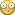forum for hobby programming:<br />https://aurelsoft.ucoz.com

#### Egil

#####April 24, 2019, 02:50:50 AM #11 Last Edit: April 24, 2019, 03:04:40 AM by Egil
I have rearranged your code so it is easier to read (posted below).

First, in CB functions must be defined before they are called. I can't find any such definitions.

Second, the last line of the program is RETURN r, th, phi[/code]. In CB you have to define these variables as UDT to be able to return them.
Another line has this contents: lamba = 3e8 / Freq. In CB powers should be written like this: lamba = 3^8 / Freq
There are several other similar instances. Think you get closer to a running program if you take a second look at the code, and redefine the variables as necessary.
Today I'm busy, but when I return home tomorrow I will have a closer look to see if I'm able to help you.

Good luck!

Egil

`'AutoDefine "Off"def win:windowdef wstyle:intdef cleft,ctop,cwidth,cheight:int :'Clientareadef run:intdef edit1:intwstyle = @SIZE|@MINBOX|@MAXBOXWINDOW win, 0,0,640,500,wstyle,0,"test", <MainLoop>GETCLIENTSIZE win,cleft,ctop,cwidth,cheightSETWINDOWCOLOR win,RGB(240,202,86)CONTROL win,"E,"",10,10,100,20,0x50800000,10" :' Edit Control'--------------------------------------------------------------------------------'DIALOG d1,0,0,295,199,0x80C80080,0,"Caption",Handler'CONTROL d1,"E,Edit1,31,44,70,20,0x50800000,1"'PatchFunction(thetaInDeg:INT, phiInDeg:INT, Freq:INT, W:INT, L:INT, h:INT, Er:FLOATPatchFunction( 90, 120, 2400, 100, 40, 5, 0.7)'-------------------------------------------------------------------------------run=1WAITUNTIL run = 0CLOSEWINDOW winEND'SUB <MainLoop>'-------------------------------------------------------------------------------------SELECT @class CASE @idcreate  centerwindow win CASE @idclosewindow run = 0 CASE @idsize GETCLIENTSIZE win,cleft,ctop,cwidth,cheight    if CONTROLEXISTS(win,10) SETSIZE win,10,40,180,30,10    endif ENDSELECTRETURN'import math'from math import cos, sin, sqrt, atan2, acosSUB PatchFunction(thetaInDeg:INT, phiInDeg:INT, Freq:INT, W:INT, L:INT, h:INT, Er:FLOAT),INT      'Taken from Design_patchr    'Calculates total E-field pattern for patch as a function of theta and phi    'Patch is assumed to be resonating in the (TMx 010) mode.    'E-field is parallel to x-axis'    W......Width of patch (m)'  L......Length of patch (m)  '  h......Substrate thickness (m)  '  Er.....Dielectric constant of substrate'    Refrence C.A. Balanis 2nd Edition Page 745'  """  lamba = 3e8 / Freq     theta_in = math.radians(thetaInDeg)  phi_in = math.radians(phiInDeg)    ko = 2 * math.pi / lamba    xff, yff, zff = sph2cart1(999, theta_in, phi_in)            :' # Rotate coords 90 deg about x-axis to match array_utils coord system with coord system used in the model.    xffd = zff    yffd = xff    zffd = yff    r, thp, php = cart2sph1(xffd, yffd, zffd)    phi = php    theta = thp    if theta = 0        theta = 1e-9                                            :'# Trap potential division by zero warning    if phi == 0:        phi = 1e-9    Ereff = ((Er + 1) / 2) + ((Er - 1) / 2) * (1 + 12 * (h / W)) ** -0.5  :'# Calculate effictive dielectric constant for microstrip line of width W on dielectric material of constant Er    F1 = (Ereff + 0.3) * (W / h + 0.264)                                  :'# Calculate increase length dL of patch length L due to fringing fields at each end, giving total effective length Leff = L + 2*dL    F2 = (Ereff - 0.258) * (W / h + 0.8)    dL = h * 0.412 * (F1 / F2)    Leff = L + 2 * dL    Weff = W                                                              :'# Calculate effective width Weff for patch, uses standard Er value.    heff = h * sqrt(Er)  ' # Patch pattern function of theta and phi, note the theta and phi for the function are defined differently to theta_in and phi_in    Numtr2 = sin(ko * heff * cos(phi) / 2)    Demtr2 = (ko * heff * cos(phi)) / 2    Fphi = (Numtr2 / Demtr2) * cos((ko * Leff / 2) * sin(phi))    Numtr1 = sin((ko * heff / 2) * sin(theta))    Demtr1 = ((ko * heff / 2) * sin(theta))    Numtr1a = sin((ko * Weff / 2) * cos(theta))    Demtr1a = ((ko * Weff / 2) * cos(theta))    Ftheta = ((Numtr1 * Numtr1a) / (Demtr1 * Demtr1a)) * sin(theta)    '# Due to groundplane, function is only valid for theta values :  0 < theta < 90  for all phi    '# Modify pattern for theta values close to 90 to give smooth roll-off, standard model truncates H-plane at theta=90.    '# PatEdgeSF has value=1 except at theta close to 90 where it drops (proportional to 1/x^2) to 0    rolloff_factor = 0.5                                                      :' # 1=sharp, 0=softer    theta_in_deg = theta_in * 180 / 3.14                                      :'  # theta_in in Deg    F1 = 1 / (((rolloff_factor * (abs(theta_in_deg) - 90)) ** 2) + 0.001)    :'  # intermediate calc    PatEdgeSF = 1 / (F1 + 1)                                                  :'  # Pattern scaling factor    UNF = 1.0006                                                              :'  # Unity normalisation factor for element pattern    if theta_in <= math.pi / 2        Etot = Ftheta * Fphi * PatEdgeSF * UNF                                :'  # Total pattern by pattern multiplication    else        Etot = 0    endifRETURN Etot'---------------------------------------------------------------------------------------------------------------------SUB sph2cart1(r, th, phi)  x = r * cos(phi) * sin(th)  y = r * sin(phi) * sin(th)  z = r * cos(th)RETURN x, y, z'------------------------------------------------SUB cart2sph1(x, y, z):  r = sqrt(x**2 + y**2 + z**2) + 1e-15  th = acos(z / r)  phi = atan2(y, x)RETURN r, th, phi`
Support Amateur Radio  -  Have a ham  for dinner!

#### aurelCB

#####April 24, 2019, 06:02:56 AM #12
Thanks Egil as always
yeah i declare functions but then i crush app and my definitions are lost ..grrr

sorry it looks that i become or rusty with CB ...

i use another compiler more often when i work on tokenizers,parsers..etc...

BUT i like CB because is simple for prototyping and experimenting..

OK i will try your code...

Quote'AutoDefine "Off"
'patch antenna fields...
declare PatchFunction(thetaInDeg:INT, phiInDeg:INT, Freq:INT, W:INT, L:INT, h:INT, Er:FLOAT)
declare sph2cart1(r:float, th:float, phi:float)
declare cart2sph1(x:int, y:int, z:int)

def win:window
def wstyle:int
def cleft,ctop,cwidth,cheight:int :'Clientarea
def run:int
def edit1:int
wstyle = @SIZE|@MINBOX|@MAXBOX
WINDOW win, 0,0,640,500,wstyle,0,"test", <MainLoop>
GETCLIENTSIZE win,cleft,ctop,cwidth,cheight
SETWINDOWCOLOR win,RGB(240,202,86)

CONTROL win,"E,"",10,10,100,20,0x50800000,10" :' Edit Control

'--------------------------------------------------------------------------------

'DIALOG d1,0,0,295,199,0x80C80080,0,"Caption",Handler

'CONTROL d1,"E,Edit1,31,44,70,20,0x50800000,1"

'PatchFunction(thetaInDeg:INT, phiInDeg:INT, Freq:INT, W:INT, L:INT, h:INT, Er:FLOAT

PatchFunction( 90, 120, 2400, 100, 40, 5, 0.7)

'-------------------------------------------------------------------------------

run=1

WAITUNTIL run = 0

CLOSEWINDOW win

END

'

SUB <MainLoop>

'-------------------------------------------------------------------------------------

SELECT @class

CASE @idcreate
centerwindow win

CASE @idclosewindow
run = 0

CASE @idsize
GETCLIENTSIZE win,cleft,ctop,cwidth,cheight

if CONTROLEXISTS(win,10)
SETSIZE win,10,40,180,30,10
endif

ENDSELECT

RETURN

'import math

'from math import cos, sin, sqrt, atan2, acos

SUB PatchFunction(thetaInDeg:INT, phiInDeg:INT, Freq:INT, W:INT, L:INT, h:INT, Er:FLOAT),INT

'Taken from Design_patchr
'Calculates total E-field pattern for patch as a function of theta and phi
'Patch is assumed to be resonating in the (TMx 010) mode.
'E-field is parallel to x-axis
'W......Width of patch (m)
'L......Length of patch (m)
'h......Substrate thickness (m)
'Er.....Dielectric constant of substrate
'Refrence C.A. Balanis 2nd Edition Page 745

'  """

lamba = 3^8 / Freq

theta_in = math.radians(thetaInDeg) :'//// i think that here I need to convert degrees to radians ?
ko = 2 * math.pi / lamba
xff, yff, zff = sph2cart1(999, theta_in, phi_in)            :'this multiple assing not wirj in any basic?

xffd = zff

yffd = xff

zffd = yff

r, thp, php = cart2sph1(xffd, yffd, zffd)

phi = php

theta = thp

if theta = 0
theta = 1e-9
endif                                            :' i am not sure that this work ?

if phi == 0:

phi = 1e-9

Ereff = ((Er + 1) / 2) + ((Er - 1) / 2) * (1 + 12 * (h / W)) ** -0.5  :'// twice multiply not work in any basic ..so what to use power?

F1 = (Ereff + 0.3) * (W / h + 0.264)                                  :'# Calculate increase length dL of patch length L due to fringing fields at each end, giving total effective length Leff = L + 2*dL

F2 = (Ereff - 0.258) * (W / h + 0.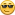dL = h * 0.412 * (F1 / F2)

Leff = L + 2 * dL

Weff = W                                                              :'# Calculate effective width Weff for patch, uses standard Er value.

heff = h * sqrt(Er)

' # Patch pattern function of theta and phi, note the theta and phi for the function are defined differently to theta_in and phi_in

Numtr2 = sin(ko * heff * cos(phi) / 2)

Demtr2 = (ko * heff * cos(phi)) / 2

Fphi = (Numtr2 / Demtr2) * cos((ko * Leff / 2) * sin(phi))

Numtr1 = sin((ko * heff / 2) * sin(theta))

Demtr1 = ((ko * heff / 2) * sin(theta))

Numtr1a = sin((ko * Weff / 2) * cos(theta))

Demtr1a = ((ko * Weff / 2) * cos(theta))

Ftheta = ((Numtr1 * Numtr1a) / (Demtr1 * Demtr1a)) * sin(theta)

'# Due to groundplane, function is only valid for theta values :  0 < theta < 90  for all phi
'# Modify pattern for theta values close to 90 to give smooth roll-off, standard model truncates H-plane at theta=90.
'# PatEdgeSF has value=1 except at theta close to 90 where it drops (proportional to 1/x^2) to 0

rolloff_factor = 0.5                                                      :' # 1=sharp, 0=softer

theta_in_deg = theta_in * 180 / 3.14                                      :'  # theta_in in Deg

F1 = 1 / (((rolloff_factor * (abs(theta_in_deg) - 90)) ** 2) + 0.001)    :'  # intermediate calc

PatEdgeSF = 1 / (F1 + 1)                                                  :'  # Pattern scaling factor

UNF = 1.0006                                                              :'  # Unity normalisation factor for element pattern

if theta_in <= math.pi / 2
Etot = Ftheta * Fphi * PatEdgeSF * UNF                                :'  # Total pattern by pattern multiplication
else
Etot = 0
endif

RETURN Etot

'---------------------------------------------------------------------------------------------------------------------

SUB sph2cart1(r, th, phi)

x = r * cos(phi) * sin(th)

y = r * sin(phi) * sin(th)

z = r * cos(th)

RETURN x, y, z

'------------------------------------------------

SUB cart2sph1(x, y, z):

r = sqrt(x**2 + y**2 + z**2) + 1e-15

th = acos(z / r)

phi = atan2(y, x)

RETURN r, th, phi
forum for hobby programming:<br />https://aurelsoft.ucoz.com

#### aurelCB

#####April 24, 2019, 06:04:32 AM #13
I put code into quote because i don't know what is wrong with code tag on this forum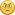forum for hobby programming:<br />https://aurelsoft.ucoz.com

#### aurelCB

#####May 01, 2019, 02:08:29 PM #14 Last Edit: May 01, 2019, 02:11:13 PM by aurelCB
Yo guys...
anyone still interested in this type of program.?

Original python program don't plot anything so i go into another direction
but i made program in another compiler for faster execution.
here is a screenshot...of not working program ..welll
but show idea how should look!
forum for hobby programming:<br />https://aurelsoft.ucoz.com